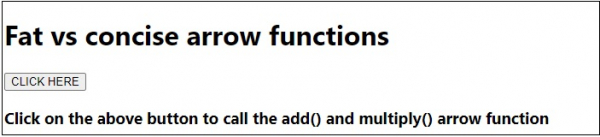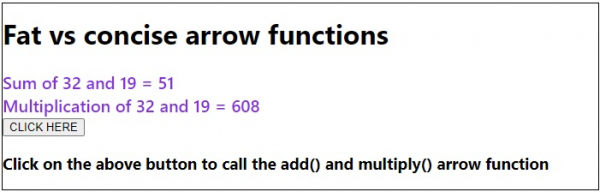# Fat vs concise arrow functions in JavaScript

The consice arrow is more stream lined form of fat arrow functions for one-line functions. If the function body only has one line of code, then there isn’t any need of curly braces {} for function body as conscie arrow functions have implicit return. Also, if there is only one parameter then that can be written without the parenthesis () but if there is no parameter then the parenthesis is necessary.

## Syntax

Fat arrow function −

let add = (a,b) =>{return a+b;}

Consice arrow function:

let add = (a,b)=>a+b;

If one parameter only −

let add = a=>a+22;

Following is the code for fat vs concise arrow functions in JavaScript −

## Example

Live Demo

<!DOCTYPE html>
<html lang="en">
<meta charset="UTF-8" />
<meta name="viewport" content="width=device-width, initial-scale=1.0" />
<title>Document</title>
<style>
body {
font-family: "Segoe UI", Tahoma, Geneva, Verdana, sans-serif;
}
.result {
font-size: 20px;
font-weight: 500;
color: blueviolet;
}
</style>
<body>
<h1>Fat vs concise arrow functions</h1>
<div class="result"></div>
<h3>Click on the above button to call the add() and multiply() arrow function</h3>
<script>
let resEle = document.querySelector(".result");
let add = (a, b) => a + b;
let multiply = (a, b) => {
return a * b;
};
resEle.innerHTML = "Sum of 32 and 19 = " + add(32, 19) + "<br>";
resEle.innerHTML =
"Multiplication of 32 and 19 = " + multiply(32, 19) + "<br>";
});
</script>
</body>
</html>

## OutputUpdated on: 17-Jul-2020

133 Views